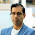## Friday, March 9, 2012

You know that Google automatically acts as a calculator, right? Type in any kind of math expression, and it automatically simplifies it in response -- including unit conversions of all sorts (very useful for that latter part, in my experience).

But here's something I discovered the other day: The calculator won't respond at all to any kind of division by zero. It won't say there's an error; it won't say it's undefined or not-a-number (NAN); it just won't trigger the calculator facility at all. It goes straight to a regular web search like it wasn't math at all. (I realized this after my first basic math class; carefully defined division and considered divide-by-zero, compared to a calculator error response, and then I asserted the same would happen in Google. Turns out that's not quite correct.)

This is true even if you try to hide the division-by-zero in some kind of very complicated expression (that's otherwise obviously math). Consider these:

And then contrast with the following:

I'm not sure if this is an oversight, or some tremendously subtle winking in-joke by our friends from Menlo Park. (Like: The calculator has to get triggered, do quite a bit of work before determing there's a divide-by-zero, and then decide to run away and hide itself from appearing.) Can you make Google Calculator admit to a divide-by-zero in any way?

1.The plot thickens: enter 0^0 and Google correctly outputs 1 (this is something which Wolfram Alpha, unbelievably and inexcusably, gets wrong, saying 'indeterminate' even while saying that sum_{i=0}^{infty}x^i/i!=e^x, which, for x=0, is inconsistent with 0^0 being "indeterminate")

I don't think it's a joke nor an oversight. When you enter 6/0, you haven't entered a meaningful mathematical question, so there is no reason to respond mathematically.

2.^ Now, my understanding is that there's legitimate debate about whether one defines 0^0 = 1 or not. As someone one said, the function a^b has a discontinuity at (0,0) no matter which way you go with it it. (Either looking at a^0 =1 mostly or 0^b = 0 mostly.)

I agree with Euler, Donald Knuth, etc., that it's best to define 0^0 = 1. But I tried arguing that we do that in our in-house custom algebra books where I teach, and it was in fact declined.

3.Next time, catch them off guard. Without any mention of 0^0, show them the series:
sum_{i=0}^{infty} x^i/i!. Ask what it converges to.
"Why, e^x, of course!"
Ask them, isn't that rather controversial?
"Nonsense, there couldn't be anything more fundamental in mathematics!"
I see... and 0!, it is 1, yes?
"Of course..."
Alright then. We are agreed, 0^0=1.

4.^ Yes, but then they can come back with what the sequence 0^b (b->0) converges to, etc.

5.It doesn't matter what the sequence 0^b converges to. There is no law that says all functions have to be continuous. x/x converges to 1 as x tends to 0, but that doesn't mean 0/0 is 1.

6.^ Well, you got me to look more closely at your argument above (3/10), and it's pretty good. But I think an opponent would say that summation is only equal to e^x for all x granted a prior definition of 0^0 = 1.

Intriguingly, this lead to me tracking down a contradiction on this point in an old calculus book of mine (Stein/Barcellos 5E, 1992). They do say of that summation, "this equation clearly holds when x = 0, since it then reduces to the equation e^0=1" (p. 631), yet elsewhere they state "0^0 is not defined" (p. S-31). Fascinating.

Anyway, I'm personally convinced that we should define 0^0=1 for purposes like these and others (binomial theorem). Thanks a bunch for the input.

7.So, you can sort of force google calculator to attempt to divide by zero: enter a non-division-by-zero expression, and then use the calculator buttons to enter, for example 8 / 0. It returns infinity though...
http://imgur.com/r3cmd

1.Yeah, that graphical calculator interface is new since I wrote that post. Slightly disappointing that it returns infinity (although that's correct in the extended reals).